# SAT II Math II : Transformations

## Example Questions

### Example Question #7 : Coordinate Geometry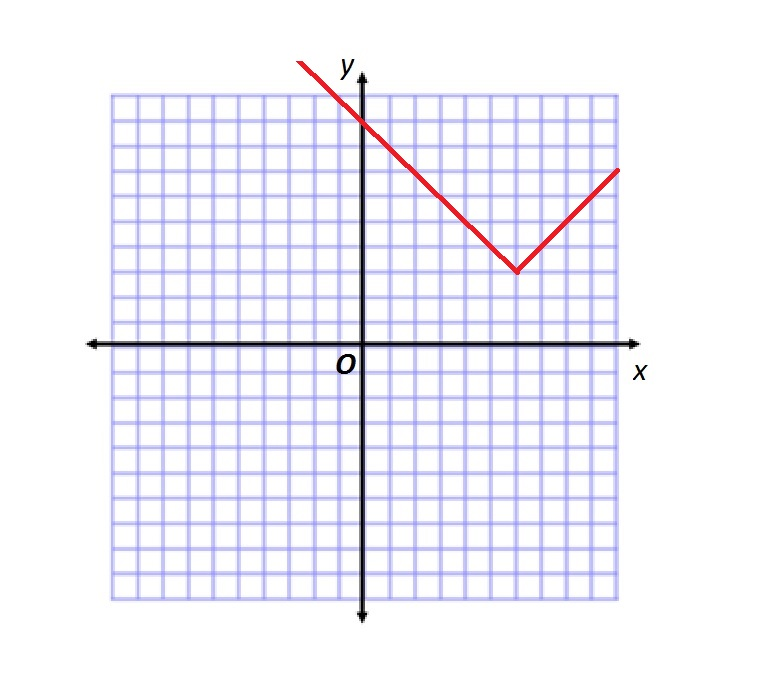Refer to the above figure.

Which of the following functions is graphed?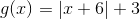The correct answer is not given among the other responses.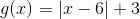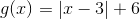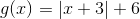Explanation:

Below is the graph of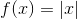: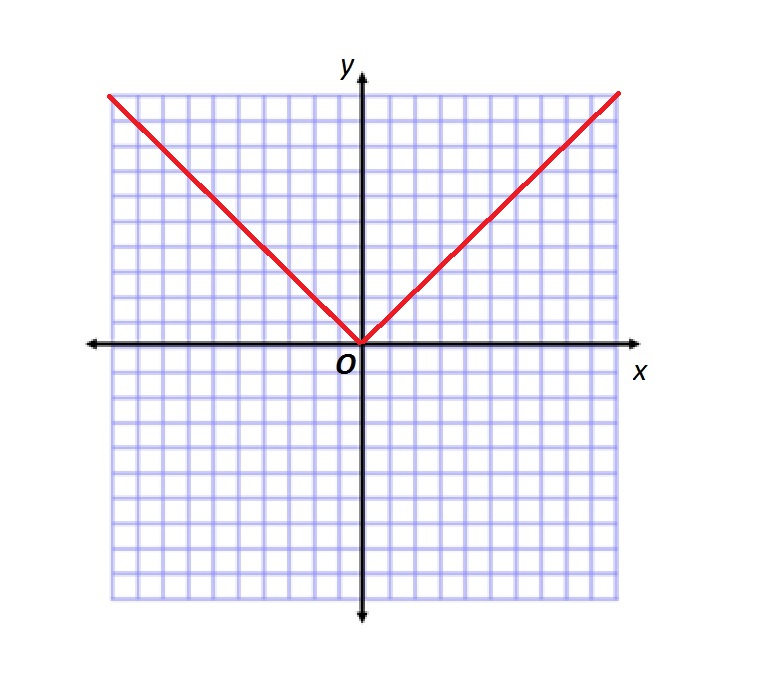The given graph is the graph ofshifted 6 units left (that is,unit right) and 3 units up.

The function graphed is therefore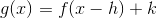where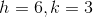. That is,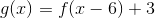### Example Question #8 : Coordinate Geometry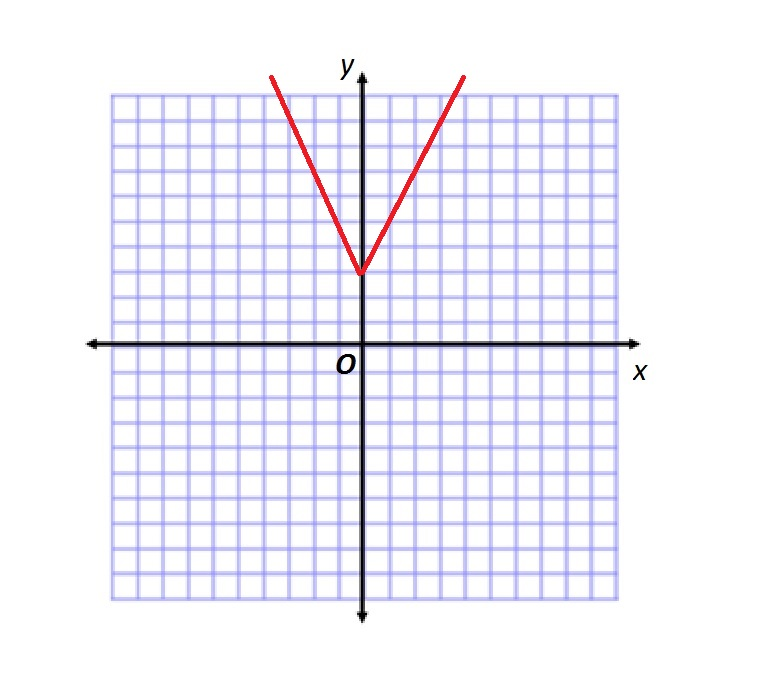Refer to the above figure.

Which of the following functions is graphed?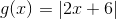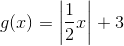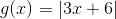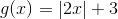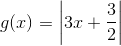Explanation:

Below is the graph of:If the graph ofis translated by shifting each point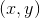to the point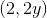, the graph of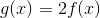is formed. If the graph is then shifted upward by three units, the new graph is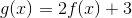Since the starting graph was, the final graph is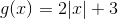, or,### Example Question #9 : Coordinate Geometry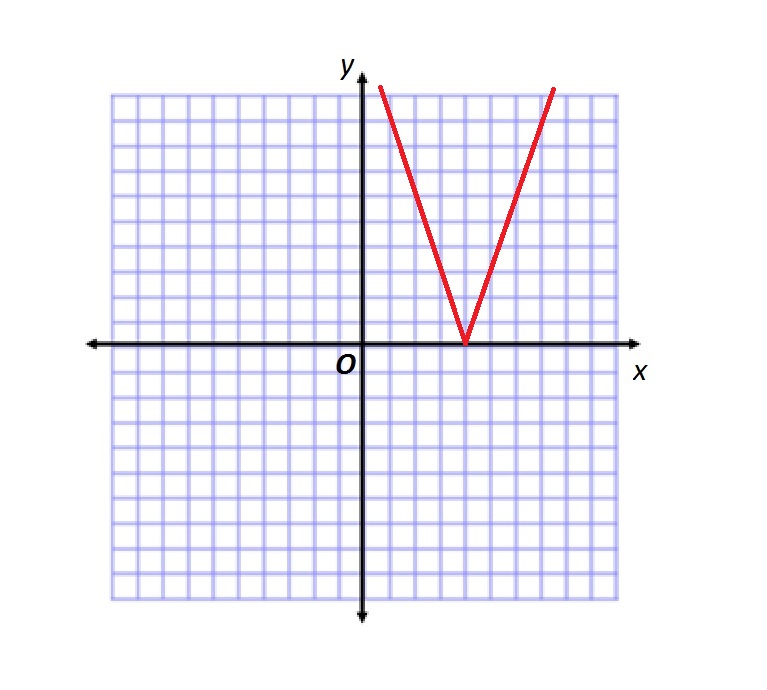Refer to the above figure.

Which of the following functions is graphed?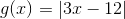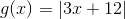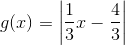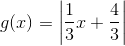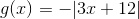Explanation:

Below is the graph of:If the graph ofis translated by shifting each pointto the point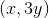, the graph of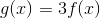is formed. If the graph is then shifted right by four units, the new graph is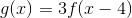Since the starting graph was, the final graph is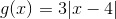, or### Example Question #1 : Transformations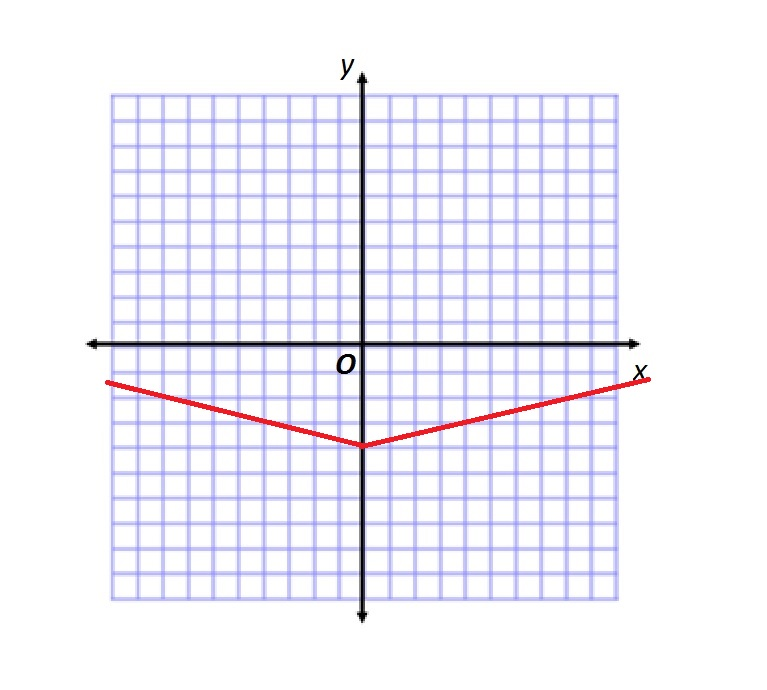Refer to the above figure.

Which of the following functions is graphed?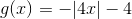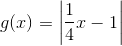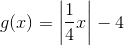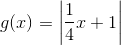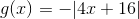Explanation:

Below is the graph of:If the graph ofis translated by shifting each pointto the point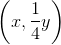, the graph of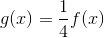is formed. If the graph is then shifted down by four units, the new graph is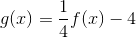.

Since the starting graph was, the final graph is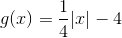, or### Example Question #1 : Transformations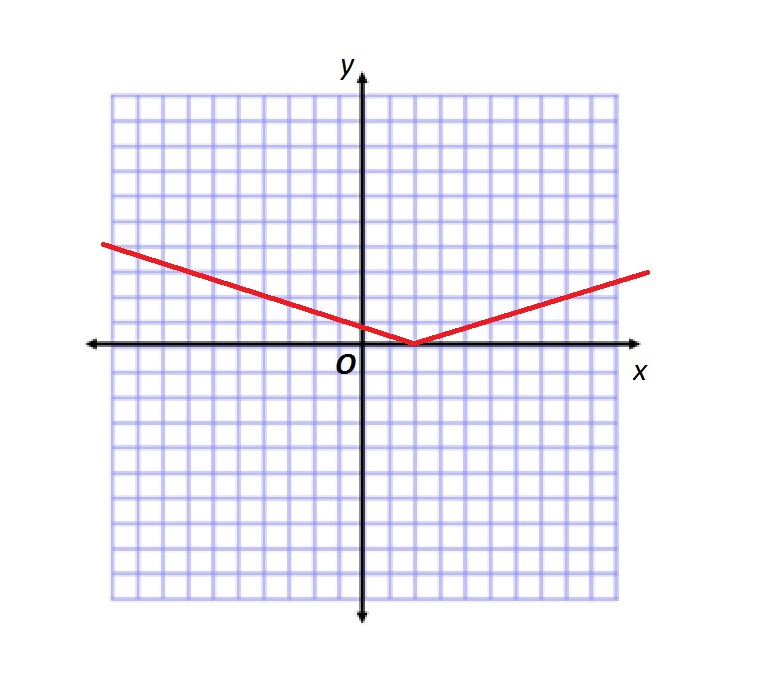Refer to the above figure.

Which of the following functions is graphed?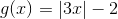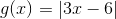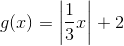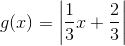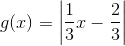Explanation:

Below is the graph of:If the graph ofis translated by shifting each pointto the point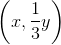, the graph of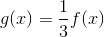is formed. If the graph is then shifted right by two units, the new graph is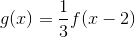Since the starting graph was, the final graph is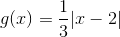, or